# NCERT Solutions(Ex: 5.7 to 5.9): Understanding Elementary Shapes Notes | Study Mathematics (Maths) Class 6 - Class 6

## Class 6: NCERT Solutions(Ex: 5.7 to 5.9): Understanding Elementary Shapes Notes | Study Mathematics (Maths) Class 6 - Class 6

The document NCERT Solutions(Ex: 5.7 to 5.9): Understanding Elementary Shapes Notes | Study Mathematics (Maths) Class 6 - Class 6 is a part of the Class 6 Course Mathematics (Maths) Class 6.
All you need of Class 6 at this link: Class 6

Exercise 5.7
Ques 1: Say true or false:
(a) Each angle of a rectangle is a right angle.
(b) The opposite sides of a rectangle are equal in length.
(c) The diagonals of a square are perpendicular to one another.
(d) All the sides of a rhombus are of equal length.
(e) All the sides of a parallelogram are of equal length.
(f) The opposite sides of a trapezium are parallel.
Ans: (a) True
(b) True
(c) True
(d) True
(e) False
(f) False

Ques 2: Give reasons for the following:
(a) A square can be thought of as a special rectangle.
(b) A rectangle can be thought of as a special parallelogram.
(c) A square can be thought of as a special rhombus.
(d) Squares, rectangles, parallelograms are all quadrilateral.
(e) Square is also a parallelogram.
Ans: (a) Because its all angles are right angle and opposite sides are equal.
(b) Because its opposite sides are equal and parallel.
(c) Because its four sides are equal and diagonals are perpendicular to each other.
(d) Because all of them have four sides.
(e) Because its opposite sides are equal and parallel.

Ques 3: A figure is said to be regular if its sides are equal in length and angles are equal in measure. Can you identify the regular quadrilateral?
Ans: A square is a regular quadrilateral.

Exercise 5.8
Ques 1: Examine whether the following are polygons. If anyone among these is not, say why?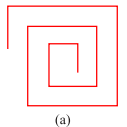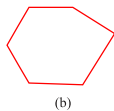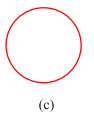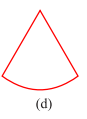Ans: (a) As it is not a closed figure, therefore, it is not a polygon.
(b) It is a polygon because it is closed by line segments.
(c) It is not a polygon because it is not made by line segments.
(d) It is not a polygon because it not made only by line segments, it has curved surface also.

Ques 2: Name each polygon: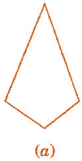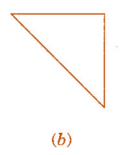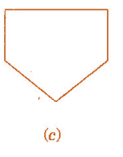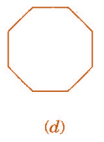Make two more examples of each of these.
(b) Triangle
(c) Pentagon
(d) Octagon

Ques 3: Draw a rough sketch of a regular hexagon. Connecting any three of its vertices, draw a triangle. Identify the type of the triangle you have drawn.
Ans: ABCDEF is a regular hexagon and triangle thus formed by joining AEF is an isosceles triangle.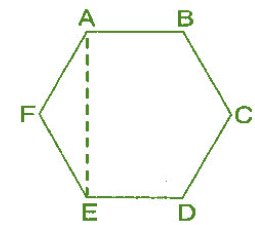Ques 4: Draw a rough sketch of a regular hexagon. Connecting any three of its vertices, draw a triangle. Identify the type of the triangle you have drawn.
Ans: ABCDEFGH is a regular octagon and CDGH is a rectangle.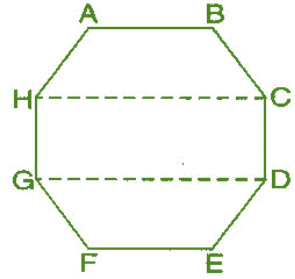Ques 5: A diagonal is a line segment that joins any two vertices of the polygon and is not a side of the polygon. Draw a rough sketch of a pentagon and draw its diagonals.
Ans: ABCDE is the required pentagon and its diagonals are AD, AC, BE and BD.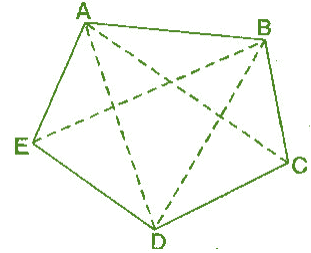Exercise 5.9
Ques 1: Match the following:

 (a) Cone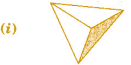(b) Sphere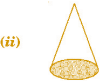(c) Cylinder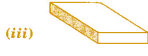(d) Cuboid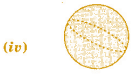(e) Pyramid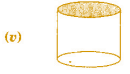Give two example of each shape.
Ans:

 (a) Cone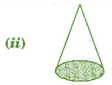(b) Sphere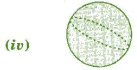(c) Cylinder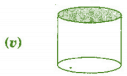(d) Cuboid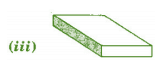(e) Pyramid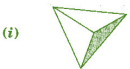Ques 2: What shape is:
(b) A brick?
(c) A match box?
Ans: (a) Cuboid
(b) Cuboid
(c) Cuboid
(d) Cylinder
(e) Sphere

The document NCERT Solutions(Ex: 5.7 to 5.9): Understanding Elementary Shapes Notes | Study Mathematics (Maths) Class 6 - Class 6 is a part of the Class 6 Course Mathematics (Maths) Class 6.
All you need of Class 6 at this link: Class 6Use Code STAYHOME200 and get INR 200 additional OFF

## Mathematics (Maths) Class 6

239 videos|245 docs|43 tests

Track your progress, build streaks, highlight & save important lessons and more!

,

,

,

,

,

,

,

,

,

,

,

,

,

,

,

,

,

,

,

,

,

;